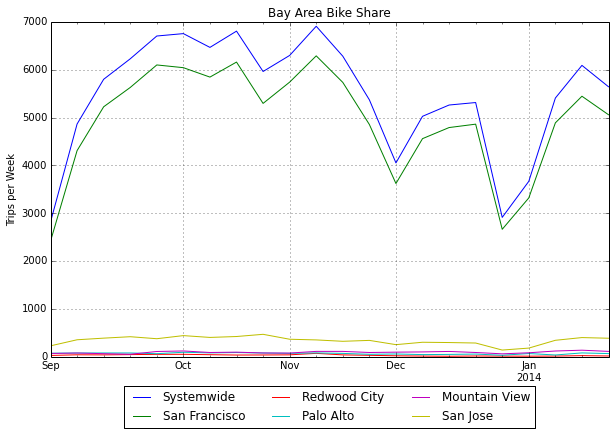In :
import numpy as np; import pandas as pd; from pandas import Series, DataFrame
df.Date = pd.to_datetime(df.Date)

In :
# df2 is the diff of the cumulative sum of df
df2 = df[['Date']]
df2['Systemwide'   ] = df['Systemwide'   ].diff()
df2['San Francisco'] = df['San Francisco'].diff()
df2['Redwood City' ] = df['Redwood City' ].diff()
df2['Palo Alto'    ] = df['Palo Alto'    ].diff()
df2['Mountain View'] = df['Mountain View'].diff()
df2['San Jose'     ] = df['San Jose'     ].diff()
df2.ix = df.ix

In :
# resample by week
df2 = df2.set_index([df2.Date])
df2 = df2.resample('W',how='sum')
# drop last incomplete week
df3 = df2[:-1]

In :
#plt.figure();
df3.plot(figsize=(10, 6))
plt.legend(loc='lower center', ncol=3, bbox_to_anchor=(0.5, -0.23))
plt.title('Bay Area Bike Share')
plt.ylabel('Trips per Week');